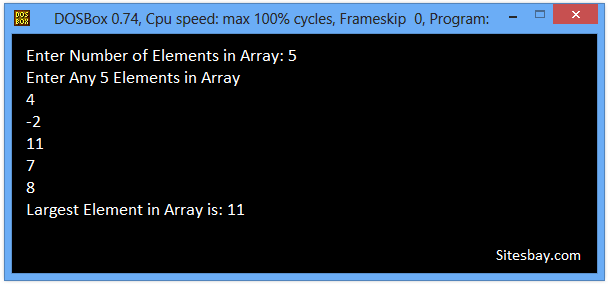# C Program to Find Largest Element in Array

## Find Largest Element in Array Program in C

To write this code we receive input from user and store in array variable after that sort array elements and find Largest element.In this Code, we will find the Largest element of array by using linear search. Given an array of N elements, we have to find the Largest Element of Array.

## Example

```Array : [9, 12, 11, 16, -14, 13, 0, -3]
Largest Element : 16
```

To understand below example, you have must knowledge of following C programming topics; String in C, for looping statements we need know For Loop in C and Array in C.

### Algorithm to Find Largest Element of Array

• First Take Number of elements as input from user. Let it be N.
• Then ask user to enter N numbers and store it in an array(lets call it input).
• Initialize one variables max with first element of input.
• Using a loop, traverse input from index 0 to N -1 and compare each element with max.
• If current element is less than max, then update max with current element.
• After array traversal max will have the Largest element.

## C Program to Find Largest Elements from Array

```#include<stdio.h>
#include<conio.h>

void main()
{
int array, count, i, largest;
clrscr();
printf("Enter Number of Elements Want in Array\n");
scanf("%d",&count);

printf("Enter Any %d count Elemnts in Array \n", count);

for(i = 0; i < count; i++)
{
scanf("%d",&array[i]);
}

largest = array;
// search num in inputArray from index 0 to elementCount-1
for(i = 0; i < count; i++)
{
if(array[i] > largest)
{
largest = array[i];
}
}

printf("Largest Element in Array is: %d",largest);

getch();
}
```

## Output

```Enter Number of Elements in Array: 5
Enter Any 5 Elements in Array
4
-2
11
7
8
Largest Element in Array is: 11
```WIFI CCTV Camera